Scientific Notation
Equations
Graphing/Solutions
1004.42* 10-3

100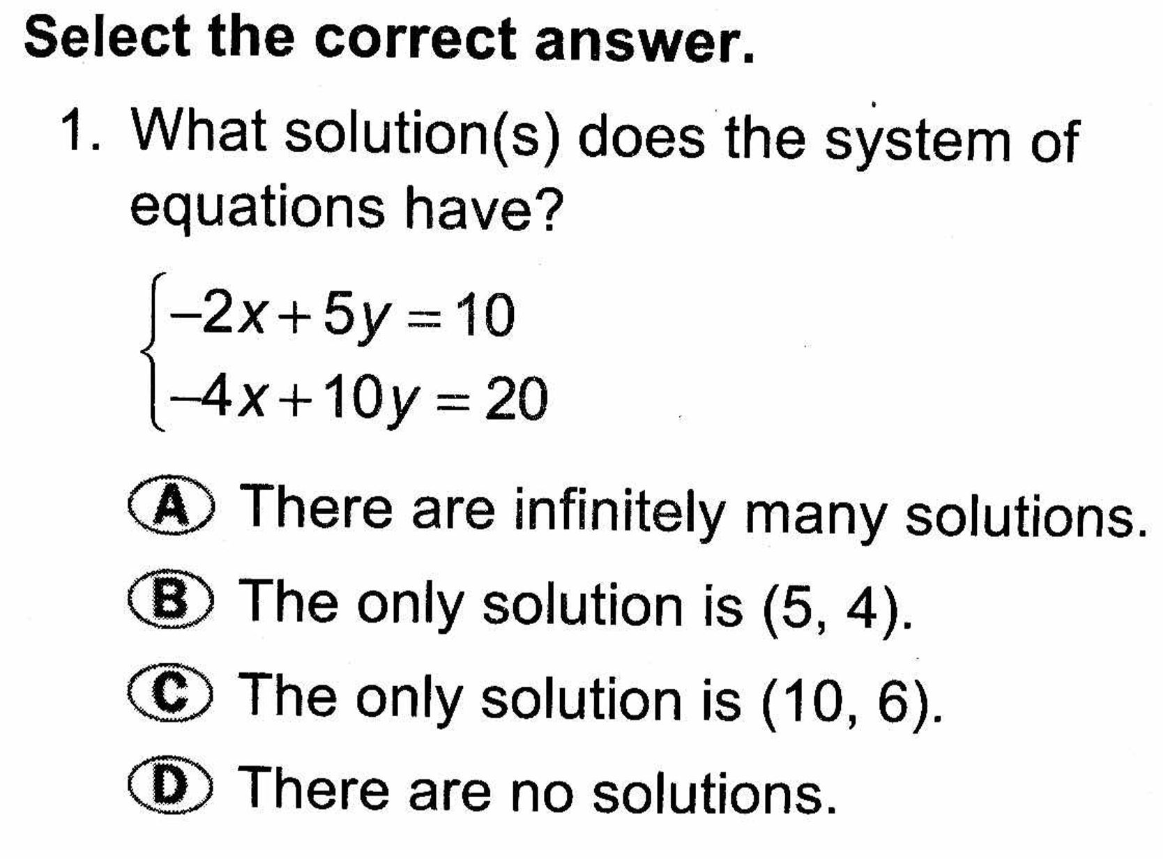A

100

Name two solutions to the equation graphed.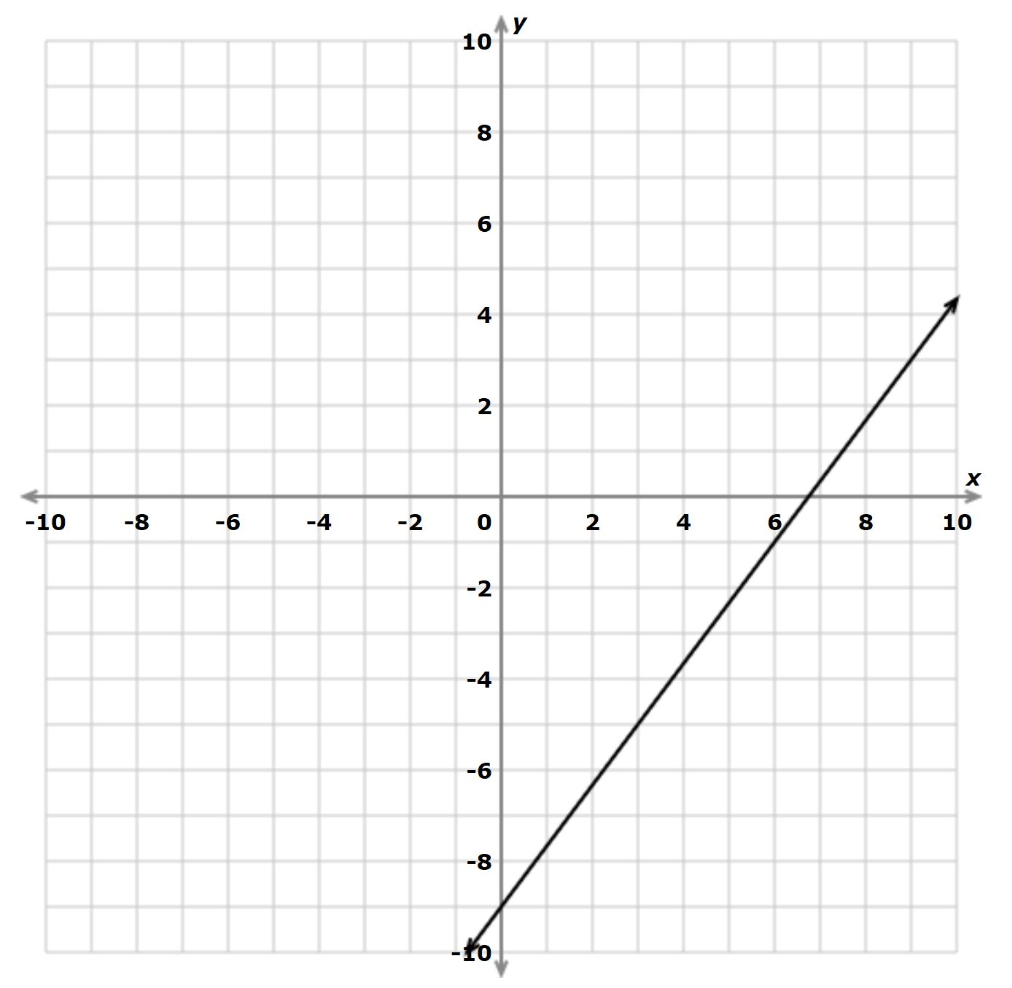(0,-9), (3,-5), (9,3)

2004.2*1021
200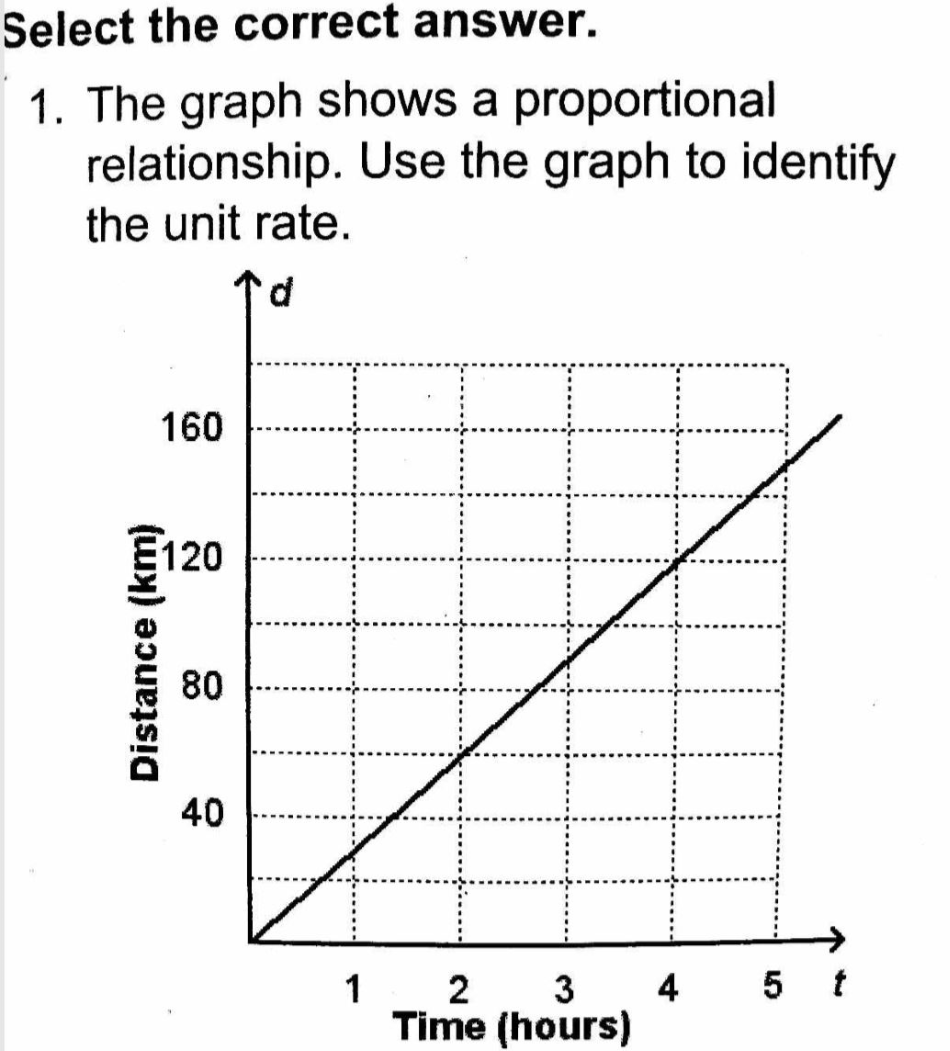60/2 =30 kph

200

What's the solution?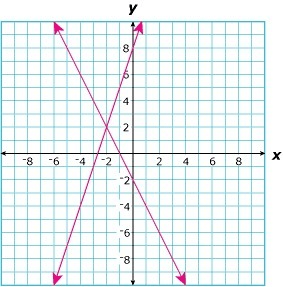(-2,2)

300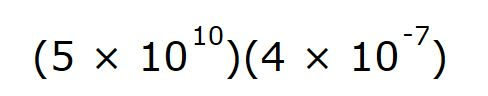2*104

300C, E

300

Determine the equations of both lines graphed.y=3x+8 and y=-2x-2

4009.6*10-38

400A

400

A system of equations is graphed below.  What is the solution?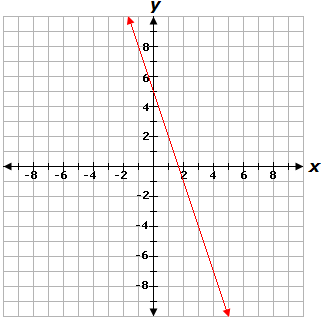Infinitely many solutions

500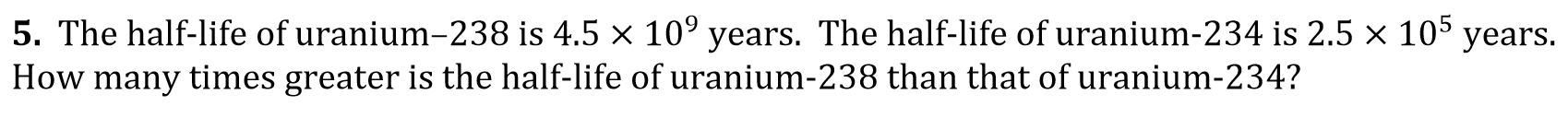1.8*104 or 18,000 times larger

500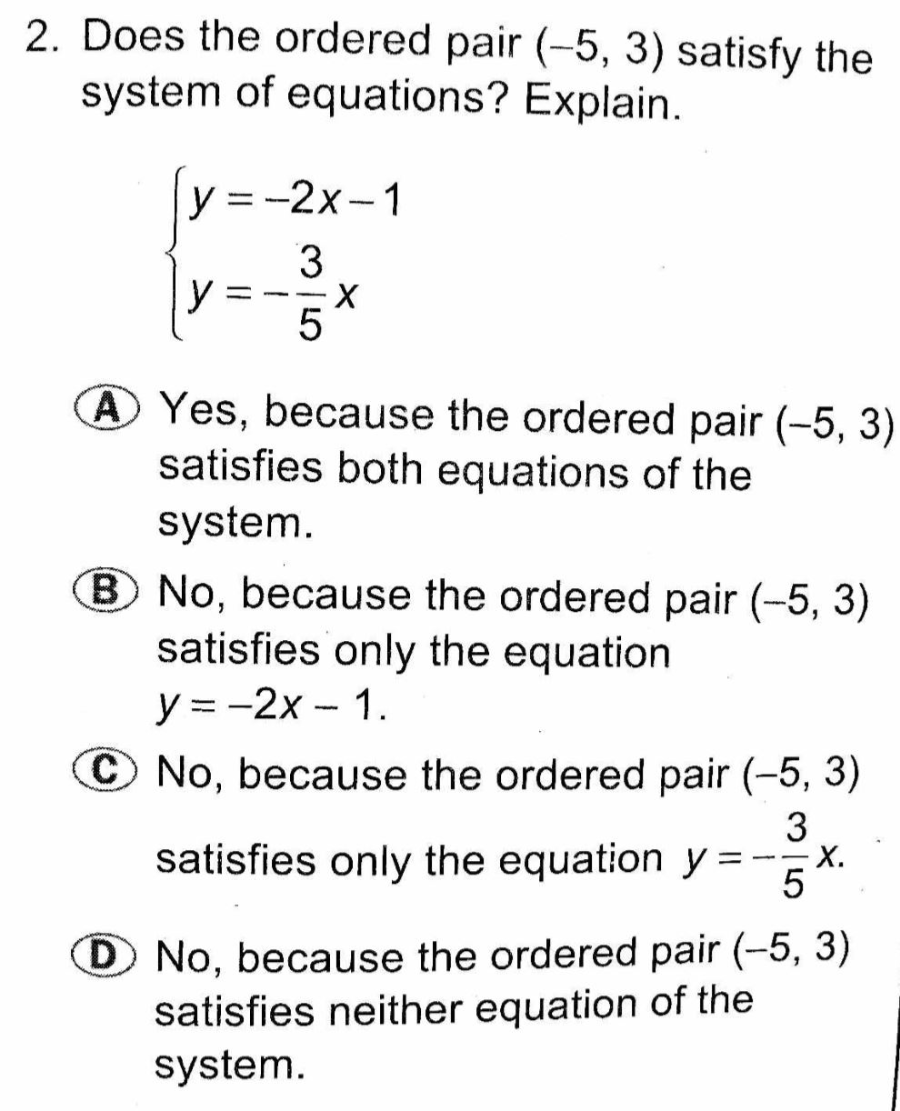C

500

What is the solution?(-1,0)

Click to zoom